# Thermodynamics with ASCEND

The ASCEND ModelLibrary includes a range of models for dealing with chemical and physical properties of materials, phase equilibrium, and reaction kinetics.

We have a brief 'HOW TO' guide for doing thermodynamics calculations with ASCEND, thanks for Pedro Vale Lima, here: howto_thermo.pdf. The following text is our partial (ongoing) transcription of that document into wikitext. The same ASCEND thermodynamics library is also covered in Chapter 11 of the ASCEND user's manual.

In addition to the methods described in this page, there are other ways of calculating thermodynamic properties using ASCEND. See FPROPS and freesteam for example.

You can access the models from the HOW TO in the ModelLibrary as models/thermodynamics_example.a4c.

## Physical property data

Physical property data from the book Reid, Prausnitz & Polling, The Properties of Gases and Liquids, 4th Ed.,McGraw-Hill, 1987, are included in the ASCEND model file models/components.a4l.

To add support for a new fluid component, it is suggested that you create your own copy of that file, and add some new code. First add your component to the supported_components list:

supported_components :== [
'hydrogen'
,'carbon_dioxide'
,(* add another one here *)
];


Then, add a CASE in the SWITCH statement to define the constants for your particular substance:

CASE 'my_new_substance':
rpp_index :== 111;



Here is the meaning of the constants that are used in the components models:

constant meaning
rpp_index Index in the Reid et al book
formula Chemical formula (optional, informational only)
subgroups Component subgroups for the UNIFAC model.
wilson_set Wilson groups for the Wilson liquid model.
mw Molecular weight
Tb Boiling-point temperature (at what pressure??)
Tc Temperature at critical point
Pc Pressure at critical point
Vc (Molar?) volume at critical point
Zc Compressibility factor at critical point.
omega Pitzer acentric factor
cpvapa..d Constants for vapour-phase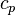$c_p$ calculation.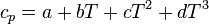$c_p = a + bT +c T^2 + d T^3$.

Hf Enthalpy of formation
Gf Gibbs free energy of formation
vpa..d Constants for vapour pressure calculation (three equations can be used)
vp_correlation Number that represents which correlation is used for calculation of vapour pressure
Hv Enthalpy of vapourisation
lden Liquid density
Tliq Temperature at which the liquid density (lden) is specified.

Finally, add the name of your new component in the check list of components model:

FOR i IN components DO

ASSERT (i IN ['hydrogen', 'carbon_dioxide' (* others here *)) == TRUE;

END FOR;


## Calculating properties for a single component

The simples state to model is the one that represents a single component in a single phase. To elaborate this model, one needs an equation of state (EOS). As there is no universal equation of state, the thermodynamic libraries in ASCEND contain several models, and leave the decision about which one to use up to the user.

The ASCEND thermodynamics library has two models for vapour components (ideal_vapour_component and Pitzer_vapor_component) and one model for liquid components (Rackett_liquid_component). All these models are similar in concept; only the EOS changes. We'll use the ideal vapour model to illustrate the main concepts.

The model defines the equations needed to calculate the molar volume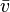${\bar v}$, enthalpy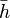${\bar h}$ and Gibbs free energy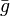${\bar g}$, based on the temperature$T$, pressure$p$ and component properties.

The first equation needed by the ideal gas model is the well known equation of state,

(1)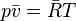$p{\bar v}={\bar R}T$

where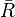${\bar R}$ is the ideal gas constant. To define${\bar h}$, one can use the constant-pressure molar heat capacity,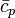${\bar c}_p$. The heat capacity is define implicitly as a polynomial function of temperature:

(2)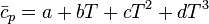${\bar c}_p = a + b T + c T^2 + d T^3$

where a, b, c, and d are defined (as cpvapa..d) in the components data file. Remembering that, for an ideal gas,${\bar h}$ is defined as

(3)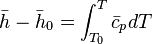${\bar h} - {\bar h}_0 = \int_{T_0}^{T} {\bar c}_p dT$

results in the following explicit equation for${\bar h}$:

(4)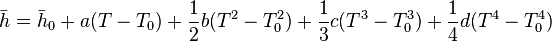${\bar h} = {\bar h}_0 + a (T-T_0) + \frac{1}{2}{b (T^2 - T_0^2)} + \frac{1}{3}c (T^3 - T_0^3) + \frac{1}{4}d (T^4 - T_0^4)$

Note that, if it were not an ideal gas,${\bar h}$ would also depend on p. Looking back again to your old thermodynamics textbook, it can then be proven that

(5)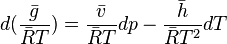$d ( \frac{\bar g}{{\bar R}T} ) = \frac{\bar v}{{\bar R}T} dp - \frac{\bar h}{{\bar R}T^2}dT$

Substitution of equations 1 and 4 on equation 5 followed by equation yields an equation for${\bar g}$ with the form

(6)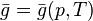$\bar g = {\bar g}(p,T)$

As a summary, this model has three equations (Eq 1, Eq 4, Eq 6), and five variables ($p$,$T$,$\bar g$,$\bar h$,$\bar v$).

### ASCEND implementation

In the thermodynamics library, we see the above code defined as follows:

MODEL ideal_vapor_component(
P WILL_BE pressure;

T WILL_BE temperature;
data WILL_BE td_component_constants;
) REFINES pure_component;

(*... *)
END ideal_vapor_component;


### Using the ASCEND single-component model

The parametric model declaration above tells you that if you want to instantiate an 'ideal_vapor_component', then you need to first declare variables for pressure 'P' and temperature 'T', as well as the thermodynamic data 'data'. Hence, we can create out little model for evaluating these properties as follows:

(* Simple example for vapour properties of water from ideal gas equation *)
REQUIRE "thermodynamics.a4l";
REQUIRE "johnpye/thermo_types.a4c";

MODEL my_props;
p IS_A pressure;
T IS_A temperature;

cd IS_A components_data(['water'],'water');
props IS_A ideal_vapor_component(p,T,cd.data['water']);

v_m ALIASES props.v;
v IS_A specific_volume;
v = Vm / props.data.mw;

rho IS_A mass_density;
rho = 1./v;
h_m ALIASES props.h;

METHODS
RUN props.default_all;
RUN props.specify;

p := 1 {atm};
T := 400 {K};

END my_props;


Using this model, ASCEND returns a calculated value of the mass-density of steam at 400 K and 1 bar as 0.54892 kg/m³. Calculation using the IAPWS-IF97 steam correlations (using freesteam via Python, specifically) gives me 0.54758 kg/m³, so the difference between the ideal gas calculation and the more rigorous correlation is not that great in this case.

Note that this model also calculates the molar Gibbs free energy and molar enthalpy for the single component.

We can now use this model to solve a simple example problem:

### Example 1

Find the change in enthalpy for water that is heated from 125 °C to 225 °C at constant pressure of 1 bar.

A model that performs this calculation is shown here:

(* model to calculate change in enthalpy from 125°C to 225 °C at 1 bar. *)
MODEL example_1;

A, B IS_A my_props;
A.p, B.p ARE_THE_SAME;

dh_m IS_A molar_energy;
dh_m = B.h_m - A.h_m;

dh IS_A specific_enthalpy;
dh = dh_m / A.props.data.mw;

METHODS

A.T := 273.15 {K} + 125 {K};

B.T := 273.15 {K} + 225 {K};

END example_1;


This model gives the result that the change in molar enthalpy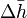$\Delta {\bar h}$ (which in the model we call 'dh_m') is equal to 3.4902 kJ/kmol. And we calculate the change in specific enthalpy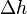$\Delta h$ to be 193.74 kJ/kg. This value compares fairly well with values from steam tables: for this process, Heywood gives$\Delta h$ = 199 kJ/kg, so the error is ~3%.

The standard model for liquid components in ASCEND (Rackett_liquid_component) has one slight difference from the vapour model present. As the enthalpy and free energy depend on knowledge of the vapor pressure, there is an additional equation with the correlation to calculate this value. You still have two degrees of freedom, but now you have six variables and four equations.

### Example 2

Calculate the temperature at which the vapour pressure for water is 1 atm.

To solve this problem, we start with the first model that was used here, but modify it to use the Rackett liquid component model instead.

(* model to calculate temperature at which vapour pressure of water is 1 atm *)
MODEL example_2;

p IS_A pressure;
T IS_A temperature;
cd IS_A components_data(['water'],'water');

props IS_A Rackett_liquid_component(p,T,cd.data['water']);

v_m ALIASES props.v;
v IS_A specific_volume;

v = v_m / props.data.mw;
rho IS_A mass_density;

rho = 1./v;

p_vap ALIASES props.VP;

METHODS
RUN props.default_all;
RUN props.specify;

p := 1 {atm};

(* reconfigure the program to solve with fixed VP and free T... *)
FREE T;

FIX p_vap;
p_vap := 1 {atm};

END example_2;


Solving this model gives the result that T = 373.14 K, which is close enough (0.014 K) to the expected value of 100 °C or 273.15 K.

## Mixtures

As with the model for single components, the model for mixtures attempts to represent the mixture molar volume, enthalpy, and Gibbs free energy.

To understand this model, we present an example. Suppose you have a mixture of two components and want to calculate the molar volume. For each component you first calculate the molar volume for each pure species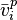${\bar v}_i^p$, where i is the index for the component, and p signifies 'pure'), using the models from the previous section. Then you calculate the molar volume for the species in the mixture,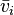${\bar v}_i$, assuming some model for the mixing. At last, the molar volume is the sum of all species contributions weighted by the molar fraction of the species in the mixture.

For example, for an ideal gas mixture, we would have the following:

(7)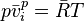$p {\bar v}_i^p = {\bar R} T$
(8)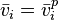${\bar v}_i = {\bar v}_i^p$
(9)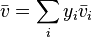${\bar v} = \sum_i {y_i {\bar v}_i}$

The first equation comes from the ideal_vapor_component model (and there is one such equation for each component in the mixture). The second equation comes from the ideal_vapor_mixture model (again, one for each component). The last one is generic for all mixture models (and is part of the phase_partials model from which all mixture models are inherited).

The same concepts apply to enthalpy and Gibbs free energy. The equations for ideal vapour mixing and mixture calculation are the following:

(10)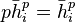$p {\bar h}_i^p = {\bar h}_i^p$
(11)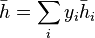${\bar h} = \sum_i {y_i {\bar h}_i}$
(12)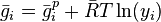${\bar g}_i = {\bar g}_i^p + {\bar R} T \ln(y_i)$
(13)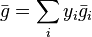${\bar g} = \sum_i {y_i {\bar g}_i}$

This model has one extra special equation whose meaning will be easier to understand after talking about phase equilibria:

(14)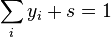$\sum_{i} {y_i + s = 1}$

This equation introduces a new variable s called slack_PhaseDisappearance. With this formulation of the molar fraction sum, when s is fixed at 0, normal calculations are performed; when s is free, if the phase ceases to exist, s will be different from zero but simulation can continue. It's a clever and important trick for multi-phase equilibria. We will return to this issue later.

To review the variables and equations present in an idea gas mixture, note that for n components, we have 3n ideal vapour component equations, and 3n + 4 mixture equations. As far as variables, we have these values for the overall mixture: p, T, h, v, g,s ; then we have these values for each component: (FIXME).

Just a minor warning: when using models where n − 1 components are fixed, usually the un-fixed one is the 'reference component'. But be sure to check this and save yourself some trouble later. Let's see this mixture code in action now.

### Example 3

Calculate the change of enthalpy for a mixture of 60% water and 40% enthanol going from 400 to 500 K.

First look at the declaration of the ideal_vapor_mixture model to work out the necessary structure. Using that, we declare our model:

MODEL water_and_ethanol;

cd IS_A components_data(['water','ethanol'], 'water');
props IS_A ideal_vapor_mixture(cd);

p ALIASES props.P;
T ALIASES props.T;

h_m ALIASES props.h_y;
METHODS
RUN props.default_self;

RUN props.specify;
RUN props.values;
p := 1 {atm};

T := 400 {K};
props.y['ethanol']&nbsp;:= 0.4;

END water_and_ethanol;

MODEL example_3;
A, B IS_A water_and_ethanol;

dh_m IS_A molar_energy;
dh_m = B.h_m - A.h_m;

METHODS

A.T := 400 {K};
B.T := 500 {K};

END example_3;


This model gives us the result that$\Delta {\bar h}$ is equal to 5.632 kJ/mol over that temperature increase.

MODEL water_and_ethanol;
cd IS_A components_data(['water','ethanol'], 'water');

props IS_A Pitzer_vapor_mixture(cd);


This gives us the slightly different result of$\Delta {\bar h}$ = 5.724 kJ/mol.

### Liquid mixtures

For mixtures involving liquids, ASCEND provides the UNIFAC mixture model, which is used together with the Rackett model for liquid properties. The number of equations in the UNIFAC model depends on the number of 'UNIFAC groups', l. For a mixture of n components, we end up with 9n + 2l + 4 equations and 10n + 2l + 6 variables, requiring us to fix n + 2 of those variables.

## Phase Equilibria

Phase equilibrium is provided by the final set of models in the ASCEND thermodynamics library. These types of models are the base for all sorts of chemical process models, starting from simple streams and working up to complex reaction and separation systems.

To model phase equilibria, one uses the thermodynamics model in the library. This model is the generic model for thermodynamics calculations, in that it can be used both for phase equilibria as well as for just single component properties or multi-phase mixtures. Of course, if you do choose to use the thermodynamics model for these simpler cases then you will be carrying around some unnecessary and redundant equations, but that's a price you might choose to pay for flexibility. The model has a generic interface and equilibrium relations that are only used for systems with at least two phases.

### Generic interface

As mixtures are models that group simple components, phase equilibria groups the same mixture at different phases. By defining γj to be the molar fraction of phase j we have the usual fraction relation:

(15)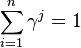$\sum_{i=1}^n{\gamma^j} = 1$

Now you must have another three equations to account for the phases:

(16)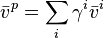${\bar v}^p = \sum_i{\gamma^i {\bar v}^i}$
(17)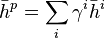${\bar h}^p = \sum_i{\gamma^i {\bar h}^i}$
(18)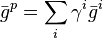${\bar g}^p = \sum_i{\gamma^i {\bar g}^i}$

where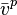${\bar v}^p$,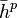${\bar h}^p$,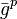${\bar g}^p$ are the mixture properties defined by equations 8, 11 and 13 for each phase.

Another group of equations (one for each component) are needed to define a global fraction for a component.

(19)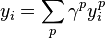$y_i = \sum_p {\gamma^p y_i^p}$

### Relative volatility model

This model has extra equations for multi-phase systems in equilibrium. The first group just equates pressures and temperature on all phases to the ones in the reference phase.

(20)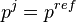$p^j = p^{ref}$
(21)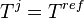$T^j = T^{ref}$

This accounts for m − 1 equations where m is the number of phases (the reference phase is excluded).

Now, recall the variable s, a.k.a. slack_PhaseDisappearance. There is one of these for each mixture. One problem with multiphase models is that models are written thinking of the existence of a phase, and if by some thermodynamics change the phase ceases to exist the equations become invalid. By using the following equation for each phase

(22)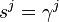$s^j = \gamma^j$

It's possible for a phase to disappear. If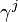$\gamma^j$ is not null, which means the phase exists, then sj must be zero and by the already-presented relation$\sum_{i}{y_i + s} = 1$ the mixture properties must be properly calculated. On the other hand, if$\gamma^j$ becomes zero then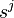$s^j$ can be relaxed and the model continues to bee solved without regard for that mixture.

The library can be used to perform just$T$ and$p$ equilibrium and fractions are computed by specification of relative volatilities. This kind of equilibrium is easier to compute and can be used as a first guess to solve the full equilibrium.

The following set of equations is a special representation of relative volatilities

(23)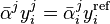${\bar \alpha}^j y_i^j = {\bar \alpha}_i^j y_i^{\mathrm{ref}}$

where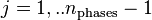$j=1,..n_{\mathrm{phases}} - 1$, and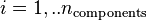$i = 1,..n_{\mathrm{components}}$. To make it easier to understand the goal of this representation let's see what these equations represent for a two phase system (V,L) of the two components (A,B).

(24)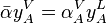${\bar \alpha} y_A^V = \alpha_A^V y_A^L$
(25)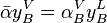${\bar \alpha} y_B^V = \alpha_B^V y_B^L$

in the relative volatility model you fix the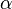$\alpha$ values and compute the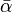$\bar \alpha$. Note that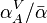$\alpha_A^V / {\bar \alpha}$ will be a K-value. Before seeing the model in action let's do some accounting. To simplify, we suppose a two-phase system, since ASCEND is not yet able to compute liquid-liquid models.

FIXME: add the equation-counting table here.

### Chemical potential equilibrium

In the full equilibrium model, the Gibbs free energy for each phase is equated. To choose full equilibrium, you should set the variable equilibrated to TRUE.

(26)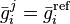${\bar g}_i^j = {\bar g}_i^{\mathrm{ref}}$

for each partial component and each phase except the reference one.

When using this model$\bar \alpha$ is fixed and equal to one and the$\alpha$'s are released and computed by equation 23. This equation becomes a computation of K-values. Now let's see the infamous accounting table for this model.

FIXME equation-counting for VL-equilibrium G-model.

Now let's look at some more examples.

### Example 4

Calculate the change of enthalpy for a 50% water, 50% ethanol being heated from 300 K to 400 K at constant atmospheric pressure.

This is a simple problem but it's useful for introducing the correct way to use the thermodynamics model.

MODEL howto_thermo_ex8 REFINES cmumodel;

cd IS_A components_data(['water','ethanol'], 'water');
pd IS_A phases_data('VL', 'ideal_vapor_mixture', 'UNIFAC_liquid_mixture',

'none');
equilibrated IS_A boolean;
phases ALIASES pd.phases;

FOR j IN phases CREATE
smt[j] IS_A select_mixture_type(cd, pd.phase_type[j]);

END FOR;
FOR j IN phases CREATE
phase[j] ALIASES smt[j].phase;

END FOR;

state IS_A thermodynamics(cd, pd,phase, equilibrated);

METHODS
(* ... *)
METHOD values;
state.P := 1 {atm};

state.T := 300 {K};
state.y['ethanol']&nbsp;:= 0.5;

equilibrated := TRUE;
END values;
(* ... *)
END howto_thermo_ex8;


Use of the thermodynamics model is slightly more complex. First, you should define the components data, then you choose the models for the phases with the phases_data model. This model's first parameter is a phase definition (it can be 'V', 'L' or 'VL'), then the name for the vapour model (or none if there's not vapour phase), and then the liquid model name. The last parameter is for a second liquid phase model when 'LL' equilibrium is implemented; for now, you should just use none.

The select_mixture_type model builds the chosen models so that they can be sent to the main thermodynamic model as a parameter. This may seem complicated at first, but as you probable will never need to change that piece of code, you don't need to worry about it too much. Just peek at the library code when you feel brave enough.

The value definitions are very simple; just notice the newly introduced equilibrated variable.

When solving multi-phase models you should always check state.slack_PhaseDisappearance. Only if it's zero the phase exists. Sometimes you can get strange results if you're looking at properties of a non-existent phase.

If you run the model, you'll get only a liquid phase and$\bar h$ = -282.351 kJ/mol. With$T$ = 400 K, you'll get$\bar h$ = -233.022 kJ/mol, and so$\Delta \bar h$ = 49.329 kJ/mol.

### Example 5

Build the VL equilibrium chart for water-ethanol at atmospheric pressure.

The model presented in the last example specifies$p$ and$T$. To build an equilibrium chart, it's better to specify$p$ and a mole fraction, and to free$T$. That way, we can be sure to have an equilibrium. The following method, added to the last example model, uses that trick. We'll also use the Pitzer vapour model, in the hope of improving accuracy.

MODEL howto_thermo_ex9 REFINES cmumodel;
cd IS_A components_data(['water','ethanol'], 'water');

pd IS_A phases_data('VL', 'Pitzer_vapor_mixture', 'UNIFAC_liquid_mixture',
'none');

equilibrated IS_A boolean;
phases ALIASES pd.phases;
(*...*)

METHOD reset_Px;
equilibrated := TRUE;
RUN state.specify;

state.T.fixed := FALSE;
state.phase['liquid1'].y['water'].fixed := TRUE;

state.P := 1 {atm};
state.y['ethanol'] := 0.5;

state.phase['liquid1'].y['water'] := 0.6;
END reset_Px;

END howto_thermo_ex9;


Now, instead of calling reset and values just run reset_Px. We'll run the model several times and build a table like the following: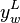$y_w^L$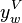$y_w^V$$T$ / [K]
0.99 0.8786 369.764
0.95 0.652 362.471
0.9 0.5517 358.80
:  :  :

When building the table, you must take some care for a phase not to disappear. You can do that by changing the variable state.phase['liquid1'].y['water'].